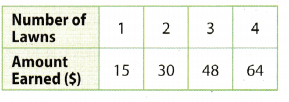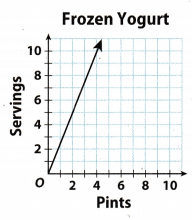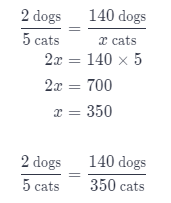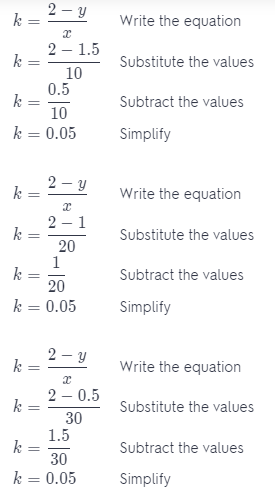Refer to our Texas Go Math Grade 7 Answer Key Pdf to score good marks in the exams. Test yourself by practicing the problems from Texas Go Math Grade 7 Module 2 Quiz Answer Key.

2.1 Unit Rates

Find each unit rate. Round to the nearest hundredth, if necessary.

Question 1.
$140 for 18 ft2 _____________ Answer: 140 ÷ 18 ≈$7.78 per ft2

Question 2.
$2.99 for 14 lb ______________ Answer: 2.99 ÷ 14 ≈$0.21 per lb

Circle the better deal in each pair. Then give the unit rate for the better deal.

Question 3.
$$\frac{\ 56}{25 \mathrm{gal}}$$ or $$\frac{\ 32.05}{15 \mathrm{gal}}$$ ____________
56 ÷ 25 = $2.24 per gal 32.05 ÷ 15 ≈$2.14 per gal
First deal is better.

Question 4.
$$\frac{\ 160}{5 \mathrm{~g}}$$ or $$\frac{\ 315}{9 \mathrm{~g}}$$ ____________
160 ÷ 5 = $32 per gram 315 ÷ 9 =$35 per gram
First deal is better.

2.2 Constant Rates of Change

Question 5.
The table shows the amount of money Tyler earns for mowing lawns. Is the relationship a proportional relationship? Why or why not?$$\frac{\text { Amount Earned }(\)}{\text { Number of Lawns }}: \frac{15}{1}=15 \quad \frac{30}{2}=15 \quad \frac{48}{3}=16$$
We can stop here because the constant are not equal. Thus, relationship is not proportional.

Question 6.
On a recent day, 8 euros were worth $9 and 24 euros were worth$27. Write an equation of the form y = kx to show the relationship between the number of euros and the value in dollars.
_____________ , where y is dollars and x is euros
$$\frac{9}{8}$$ = 1.125
$$\frac{27}{24}$$ = $$\frac{9}{8}$$ = 1.125
k = 1.125
y = 1.125 x

2.3 Proportional Relationships and Graphs

Question 7.
The graph shows the number of servings in different amounts of frozen yogurt listed on a carton. Write an t equation that gives the number of servings y in x pints.The relationship is obviously proportional. Thus. we only need 1 point to determine the constant.
$$\frac{5}{2}$$ = 2.5
y = 2.5x

Question 8.
A refreshment stand makes 2 large servings of frozen yogurt from 3 pints. Add the line to the graph and write 2 its equation.
$$\frac{2}{3}$$ servings per pint Equation: y = $$\frac{2}{3}$$ x.Essential Question

Question 9.
How can you use rates to determine whether a situation is a proportional relationship?
Rates need to be the equal for a relationship to be proportional.

Texas Go Math Grade 7 Module 2 Mixed Review Texas Test Prep Answer Key

Selected Response

Question 1.
Kori spent $46.20 on 12 gallons of gasoline. What was the price per gallon? A.$8. 35
B. $3.85 C.$2.59
D. $0.26 Answer: B.$3.85

46.20 ÷ 12 = 3.85$per gallon Question 2. A rabbit can run 35 miles per hour. A fox can run 21 miles in half an hour. Which animal is faster, and by how much? A. The rabbit is faster by 7 miles per hour. B. The fox is faster by 7 miles per hour. C. The rabbit is faster by 14 miles per hour. D. The fox is faster by 14 miles per hour. Answer: C. The rabbit is faster by 14 miles per hour. The rabbit runs can run more miles per hour, that means he is faster. 35 – 21 = 14 The rabbit is faster by 14 miles per flour. Question 3. A pet survey found that the ratio of dogs to cats is $$\frac{2}{5}$$. Which proportion shows the number of dogs if the number of cats is 140?Answer:Question 4. What is the cost of 2 kilograms of flour, if 3 kilograms cost$4.86 and the unit price for each package of flour is the same?
A. $0.81 B.$2.86
C. $3.24 D.$9.72
C. 3.24

First, notice that 2 is equal to $$\frac{2}{3}$$ of 3. Thus, price of 2 kilograms will be $$\frac{2}{3}$$ of the price of 3 kilograms.
4.86 × $$\frac{2}{3}$$ = 3.24
The price of 2 kilograms of flour is \$3.24.

Question 5.
One gallon of paint covers about 450 square feet. How many square feet will 1.5 gallons of paint cover?
A. 300 ft2
B. 451.5 ft2
C. 675 ft2
D. 900 ft2
C. 675 ft2

First, notice that 1.5 is equal to 1 × 1.5. Thus, 1.5 galLons of paint wilL cover 1.5 × 450 (how much 1 gallon covers).
1.5 × 450 = 675
1.5 gallons will cover 675 ft2.

Question 6.
The graph shows the relationship between the late fines the library charges and the number of days late.What is an equation for the relationship?
A. y = 0.25x
B. y = 0.40x
C. y = 0.50x
D. y = 0.75x
A. y = 0.25x

First, notice that points form a line. Thus, we will need only one point to determine the equation of the relationship.
y ÷ x = 0.5 ÷ 2 = 0.25
The equation of a relationship is y = 0.25x.

Gridded Response

Question 7.
School is 2 miles from home along a straight road, The table shows your distance from home as you walk home at a constant rate. Give the constant of proportionality as a decimal.k = $$\frac{2-y}{x}$$